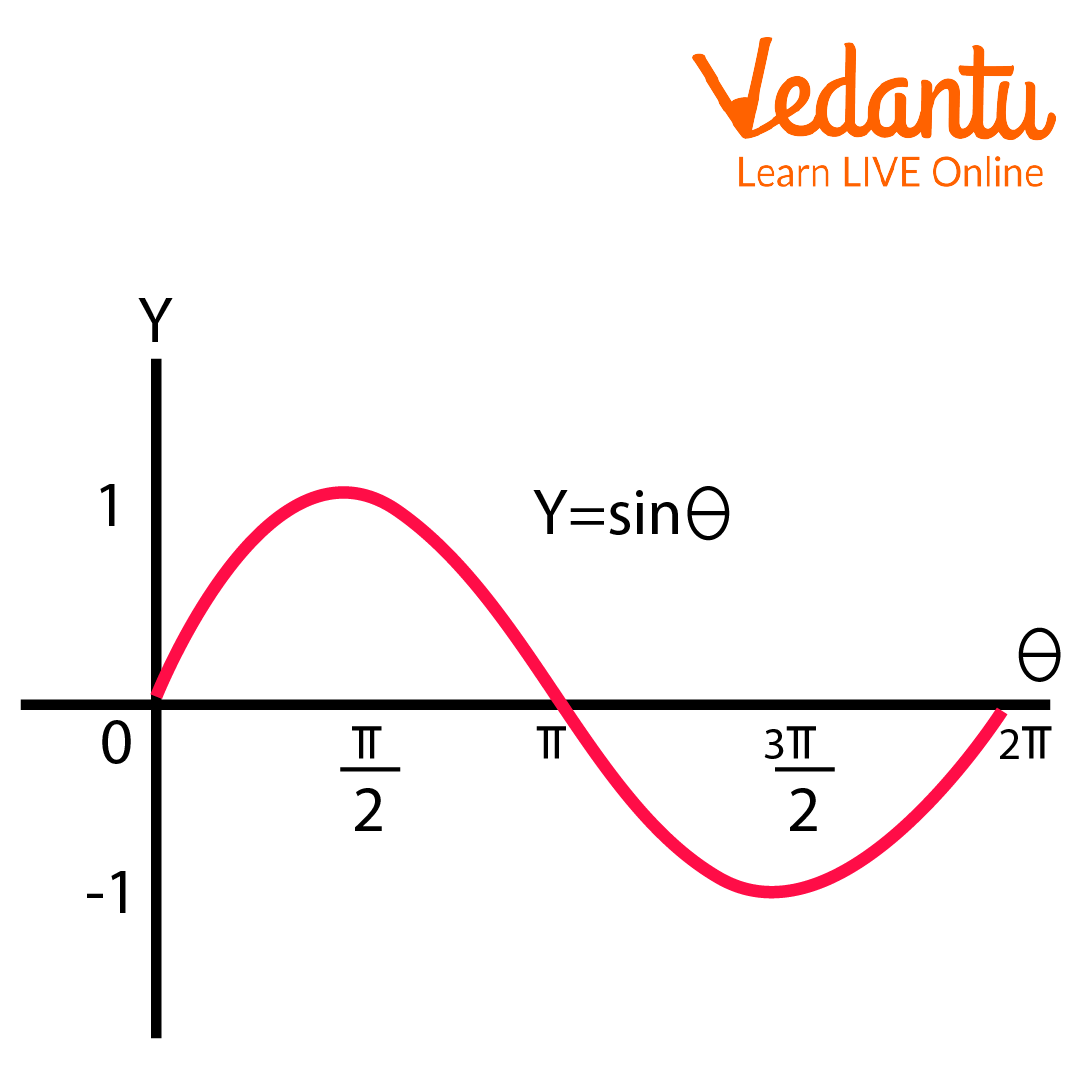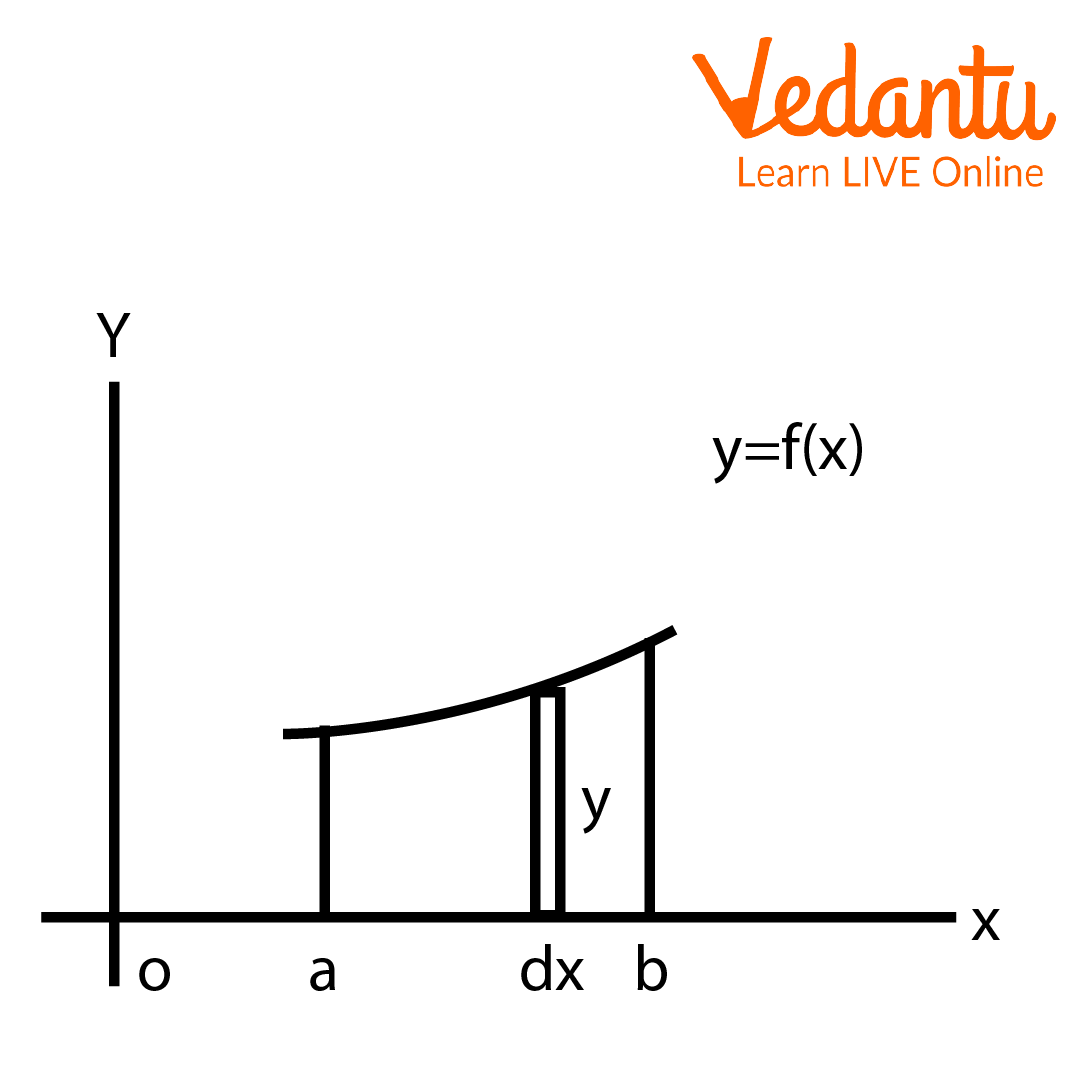Courses
Courses for Kids
Free study material
Offline Centres
More

# Reduction TheoremLast updated date: 28th Nov 2023
Total views: 114.6k
Views today: 2.14k## An Introduction to Reduction

Reduction Theorem Formulas help us in reducing the tedious work of calculation, the lengthy integrations, and reduce our mathematical computational work. These formulas are used to compute the higher order integrations. In this article, we will discuss the formulas for various types of expressions.

## Reduction Theorem/Reduction for Basic Exponential Expressions

$\int x^{n} \cdot e^{m x} \cdot d x=\dfrac{1}{m} \cdot x^{n} \cdot e^{m x}-\dfrac{n}{m} \int x^{n-1} \cdot e^{m x} \cdot d x$

## Statement of Reduction Theorem/Reduction for Logarithmic Expressions

• $\int \log ^{n} x \cdot d x=x \log ^{n} x-n \int \log ^{n-1} x \cdot d x$

• $\int x^{n} \log ^{m} x \cdot d x=\dfrac{x^{n+1} \log ^{m} x}{n+1}-\dfrac{m}{n+1} \int x^{n} \log ^{m-1} x \cdot d x$

## Reduction Theorem/Reduction for Trigonometric Functions

• $\int \sin ^{n} x \cdot d x=-\dfrac{1}{n} \sin ^{n-1} x \cdot \cos x+\dfrac{n-1}{n} \int \sin ^{n-2} x \cdot d x$

• $\int \cos ^{n} x \cdot d x=\dfrac{1}{n} \cos ^{n-1} x \cdot \sin x+\dfrac{n-1}{n} \int \cos ^{n-2} x \cdot d x$

• $\int \sin ^{n} x \cdot \cos ^{m} x \cdot d x=\dfrac{\sin ^{n+1} x \cdot \cos ^{m-1} x}{n+m}+\dfrac{m-1}{n+m} \int \sin ^{n} x \cdot \cos ^{m-2} x \cdot d x$

• $\int \tan ^{n} x \cdot d x=\dfrac{1}{n-1} \cdot \tan ^{n-1} x-\int \tan ^{n-2} x \cdot d x$

## Statement of Reduction Theorem/Reduction for Algebraic Expressions

$\int \dfrac{x^{n}}{a x^{n}+b} \cdot d x=\dfrac{x}{a}-\dfrac{b}{a} \int \dfrac{1}{a x^{n}+b} \cdot d x$Sin function

## Properties of Reduction

• Reduction Formulas are used to evaluate higher order integrals that cannot be evaluated otherwise.

• Reductions Formulas are applicable across a wide range of variables such as exponential, logarithmic, algebraic, trigonometric, etc.Area Under the Curve

## Solved Examples

1. Find the integral of $\sin ^{5 x}$.
Ans:
Apply reduction formula:

$\int \sin ^{n} x \cdot d x=-\dfrac{1}{n} \sin ^{n-1} x \cdot \cos x+\dfrac{n-1}{n} \int \sin ^{n-2} x \cdot d x \int \sin ^{5} x \cdot d x \\$

$\Rightarrow-\dfrac{1}{5} \cdot \sin ^{4} x \cdot \cos x+\dfrac{4}{5} \int \sin ^{3} x \cdot d x \\$

$\Rightarrow-\dfrac{1}{5} \cdot \sin ^{4} x \cdot \cos x+\dfrac{4}{5}\left(\int \dfrac{(3 \sin x-\sin 3 x)}{4} \cdot d x\right) \\$

$\Rightarrow-\dfrac{1}{5} \cdot \sin ^{4} x \cdot \cos x+\dfrac{1}{5}\left(3 \int \sin x \cdot d x-\int \sin 3 x \cdot d x\right) \\$

$\Rightarrow-\dfrac{1}{5} \sin ^{4} x \cdot \cos x+\dfrac{1}{5}\left(-3 \cos x+\dfrac{\cos 3 x}{3}\right) \\$

$\Rightarrow-\dfrac{1}{5} \cdot \sin ^{4} x \cdot \cos x-\dfrac{3 \cos x}{5}+\dfrac{\cos 3 x}{15}$

Therefore, we have

$\Rightarrow \int \sin ^{5} x \cdot d x=-\dfrac{1}{5} \cdot \sin ^{4} x \cdot \cos x-\dfrac{3 \cos x}{5}+\dfrac{\cos 3 x}{15}$

2. Evaluate the integral of $x^{3} \log ^{2} x$.
Ans:
Applying the reduction formula:

$\int x^{n} \log ^{m} x \cdot d x=\dfrac{x^{n+1} \log ^{m} x}{n+1}-\dfrac{m}{n+1} \int x^{n} \log ^{m-1} x . \\$

$\int x^{3} \log ^{2} x \cdot d x=\dfrac{x^{4} \log ^{2} x}{4}-\dfrac{2}{4} \int x^{3} \log x \cdot d x \\$

$\Rightarrow \dfrac{x^{4} \log ^{2} x}{4}-\dfrac{1}{2}\left(\dfrac{x^{4} \log x}{4}-\dfrac{1}{4} \cdot \int x^{3} \cdot d x\right) \\$

$\Rightarrow \dfrac{x^{4} \log ^{2} x}{4}-\dfrac{x^{4} \log x}{8}+\dfrac{x^{4}}{32}+C$

Therefore, we have

$\Rightarrow \int x^{3} \log ^{2} x \cdot d x=\dfrac{x^{4} \log ^{2} x}{4}-\dfrac{x^{4} \log x}{8}+\dfrac{x^{4}}{32}+C$

3. Evaluate $\int_{0}^{2 a} x^{2} \sqrt{2 a x-x^{2}} d x$.
Ans:

Put $x=2 \cos ^{2} \theta$.

So, $d x=-4 a \cos \theta \sin \theta d \theta$.

At $x=0,2 \operatorname{acos}^{2} \theta=0$ and so $\theta=\dfrac{\pi}{2}$.

When $x=2 a, 2 a \cos ^{2} \theta=2 a$ and so $\theta=0$.

Hence, we have

$\Rightarrow I=\int_{0}^{2 a} x^{2} \sqrt{2 a x-x^{2}} d x$

$\Rightarrow \int_{\dfrac{\pi}{2}}^{0} 4 a^{2} \cos ^{2} \theta \sqrt{4 a^{2} \cos ^{2} \theta-4 a^{2} \cos ^{4} \theta}(-4 a \cos \theta \sin \theta) d \theta$

$\Rightarrow \int_{0}^{\dfrac{\pi}{2}} 4 a^{2} \cos ^{2} \theta 2 a \cos \theta \sin \theta(4 a \cos \theta \sin \theta) d \theta$

$\Rightarrow 32 a^{4} \int_{0}^{\dfrac{\pi}{2}} \cos ^{4} \theta \sin ^{2} \theta d \theta$

$\Rightarrow 32 a^{4} \times \dfrac{1}{6} \times \dfrac{3}{4} \times \dfrac{1}{2} \times \dfrac{\pi}{2}$

$\Rightarrow \pi a^{4}$

## Conclusion

In the article, we have discussed the detailed proof of Reduction Formulas and their applications. They are very helpful and reduce our computational work. So, reduction formulas are very important and can be derived using simple integration formulas; however, the proof is not important for us, so we need to remember all the formulas used to compute higher-order integrals.

## Important Points to Remember

All the Reduction Formulas are important and are to be remembered.

## FAQs on Reduction Theorem

1. What do you mean by integration?

Integration in Mathematics is a fundamental tool of calculus and is also referred to as an anti-derivative of a function. Integration can be calculated for the values which are collections of small data and cannot be computed for values that occur singularly. So, integration is defined as the summation of discrete data. There are two types of integration: Definite integrals and indefinite integrals. The functions defined over a fixed interval are definite integrals; otherwise, they are termed indefinite integrals.

2. What do you mean by a reduction in Calculus?

Reduction in calculus over integration is defined as reducing a higher order derivative in terms of lower order integrals to compute the value in easier integrals problems. For the reduction of integration, we have different reduction formulas for different types of functions. The reduction of integrals can be done for single-valued and multi-valued functions. Reduction formulas in calculus make integration easier and saves lots of computational time and energy.

3. Are there reduction formulas for hyperbolic trigonometric functions?

Hyperbolic Trigonometric functions are not to be confused with Trigonometric functions. The trigonometric functions are associated with the imaginary part of the exponential function, while the hyperbolic trigonometric functions are associated with the real part of the exponential function. So, we have reduction formulas for hyperbolic trigonometric functions. As we have reduction formulas for exponential functions and hyperbolic trigonometric functions are real parts of exponential functions, so we can easily derive reduction formulas for such functions also.